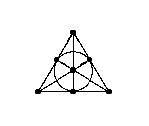E D T

# Encyclopaedia of DesignTheory: STS

 General Partition Incidence Array Experimental Other designs Math properties Stat properties Server External Bibliography Miscellaneous

# Steiner triple systems

A Steiner triple system consists of a set X of n points, and a collection B of subsets of X called blocks or triples, such that each block contains exactly 3 points, and any two points lie together in exactly one block. We abbreviate "Steiner triple system" to STS; the number n of points is its order.

We regard an STS of order 3 or less as trivial.

In other words, a non-trivial STS is a 2-(n,3,1) design, that is, the special case t=2, k=3, l=1 of a t-design.

One of the oldest results in design theory is Kirkman's existence theorem for Steiner triple systems: a STS of order n exists if and only if n is congruent to 1 or 3 mod 6. (Kirkman's proof predated Steiner's proposing the problem by several years; by rights they should be called "Kirkman triple systems", but this term is used for a special case, as we will see.) Numbers n of this form are called admissible orders for Steiner triple systems.

Thus, the smallest admissible order of a non-trivial STS is 7. There is up to isomorphism a unique STS of order 7. It can be represented in various nice ways:

• A cyclic representation: the point set is {0,1,...,6} (the integers mod 7), and the triples are the set {1,2,4} of quadratic residues mod 7 and its cyclic shifts ({2,3,5}, {3,4,6}, ..., {0,1,3}).
• A binary representation: the points are all triples of binary digits except 000, and the blocks are all sets of three such triples with sum zero (for example, {110,101,011}).
• Interpreting the above as integers in base 2, we obtain the Nim representation: the point set is {1,2,...,7}, and the blocks are {1,2,3}, {1,4,5}, {1,6,7}, {2,4,6}, {2,5,7}, {3,4,7}, {3,5,6}. (These are all the winning positions in Nim consisting of three piles with at most seven counters in each.)
• Here is the famous picture of this system:This system is also known as the projective plane of order 2, or the Fano plane. (Paradoxically, Fano had studied projective planes not containing this configuration!)

A Kirkman triple system is a resolvable Steiner triple system; that is, one whose blocks can be partitioned into "parallel classes" so that each point lies on a unique block of each parallel class. Kirkman's "schoolgirl problem" asked for the set of orders of such systems. Clearly the order must be divisible by 3.

For n=9, there is a unique Steiner triple system, which is a Kirkman system; it is otherwise known as the affine plane of order 3. Taking the points as the cells of a 3 x 3 matrix, the blocks are the rows, the colummns, and the six triples corresponding to the terms in the determinant of the matrix. Another representation takes the points to be the pairs of elements from the integers mod 3; the blocks are all sets of three distinct pairs having sum zero.

Explicitly, the point set is {1,2,...,9}, and the blocks (grouped in parallel classes) are {1,2,3}, {4,5,6}, {7,8,9}; {1,4,7}, {2,5,8},{3,6,9}; {1,5,9}, {2,6,7}, {3,4,8}; {1,6,8}, {2,4,9}, {3,5,7}.

More than a century after the problem was posed by Kirkman, it was solved by Ray-Chaudhuri and Wilson: a Kirkman triple system of order n exists for all n congruent to 3 mod 6.

Peter J. Cameron
16 April 2002